## Quiz 11 : Bond Pricing and Amortization BondsLooking for Accounting Homework Help?# Quiz 11 : Bond Pricing and Amortization Bonds

Bond: It is a debt instrument or a debt security in which an investor lends money to the company, which is to be repaid by the company at a later date, along with the interest and principal. The date on which the amount taken in exchange of a bond is repaid on the due date, the date is called the maturity date. Straight line amortization: It is the method, where the premium on the bond is amortized and it allocates equal amount of interest over each accounting period throughout its life. The amortization of premium records the interest expense associated with the bond equally till the maturity period. Calculate the Issuance value of the bond as follows:Compute the amortization schedule as per straight line method: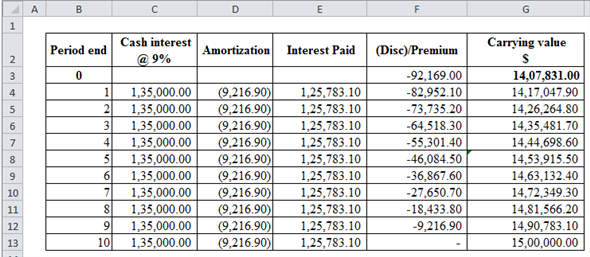Formulas for the above table are shown below: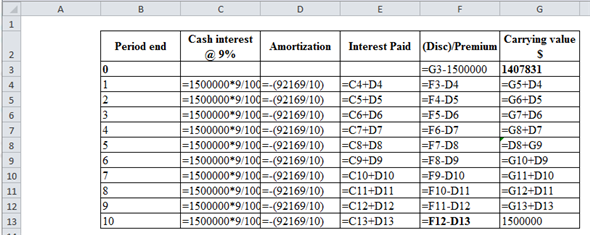Compute the amortization schedule as per effective interest rate method: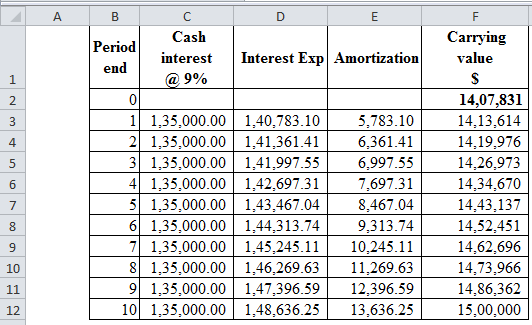Calculations are shown as follows: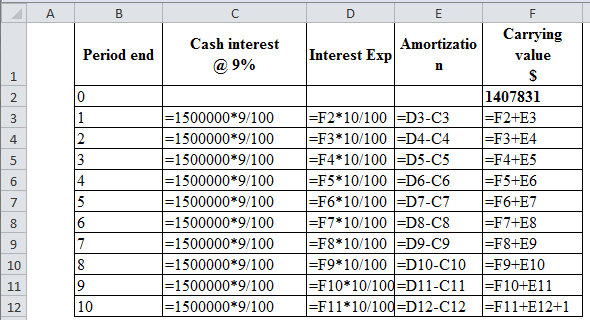When working with a spreadsheet, a formula can be used to determine the net present value of bonds. To correctly write it, one must understand the different parts that make up the formula. An example of a formula to calculate net present value is: =NPV(E10,C60:C71)+NPV(E10,D60:D710 To understand the formula, let's break it down into two parts. a. The first part is =NPV(E10,C60:C71). In this example, E10 is the cell that has the effective interest rate. C60:C71 is the annual interest. So, this will calculate the net present value of cells C60:C71 with the interest rate in cell E10. b. The second part is +NPV(E10,D60:D71). Again, E10 is the effective interest rate and cells D60:D71 shows the bond maturity. So, this part of the formula will calculate the net present value of D60:D71 with the interest rate in E10. This formula will add the net present value of the annual interest to the net present value of the bond maturity.

The formulas will be entered to compute the bond issue price, and the amortization schedule using both straight line method and effective interest method. The spreadsheet is given below: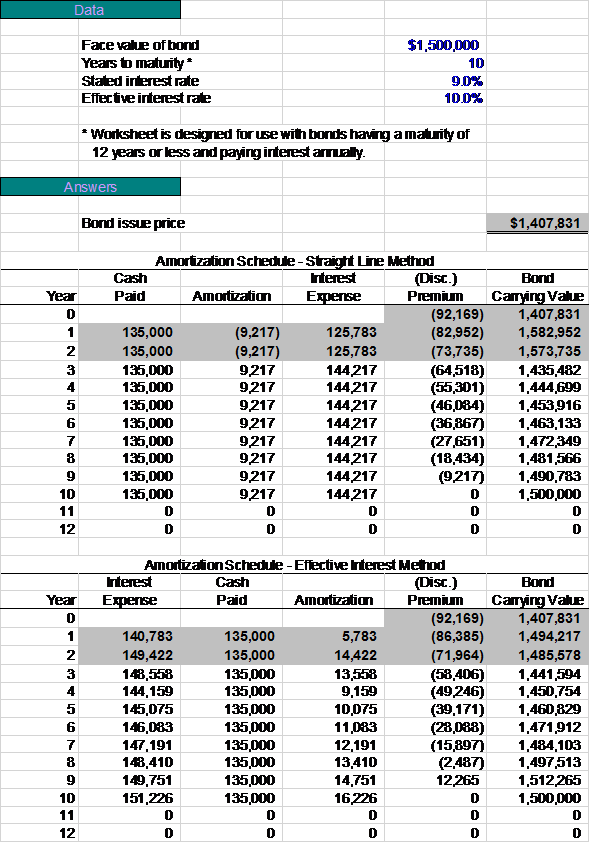In straight line method, the same amount is paid as interest and amortized each year. At the end of 10 year maturity, the value is \$1,500,000. The effective interest method uses interest based on the face value of the bond but again at the 10 year maturity, the value is \$1,500,000.